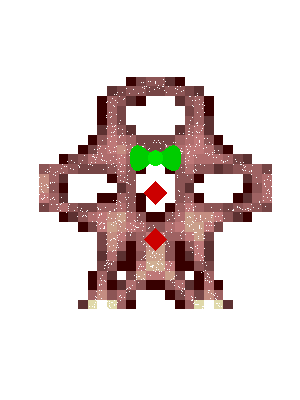# Ginger Plot Winner

If you've been following the comments from my recent post on chaotic maps, you know that there were several entries to the challenge of coming up with an interesting visualization. The entries are each interesting, displaying schemes, including an animation, and extra information placed on the plots in various ways. I have conferred with a few colleagues here and we have chosen the contribution from Rafael Oliveira as the winning entry. Why? Because the code is interesting, he made interesting use interesting of some functions, the and the plot is cute (at least for rng(42).

### Contents

gingerRHere's a comment from Rafael for this submission:

I decided to make a pixelated gingerbread man with the output of the chaotic map, using a lemniscate of Gerono as his green bow tie :)

### The Code

I took the liberty of adding a small number of comments to Rafael's code to highlight some parts of the plot.

type gingerR
function gingerR
%function pixelatedGBM from Rafael
rng(42) % could comment this out to get other "men"
% scale and rotate our gingerbread man
r = [x y] * [cosd(135) -sind(135); sind(135) cosd(135)];
minR = min(r); maxR = max(r);
r = (r - repmat(minR,size(r,1),1))./repmat(maxR-minR,size(r,1),1);
r(:,2) = r(:,2).^1.5;

% create a pixel representation of it
N = 25;
b = linspace(0,1,N);
dif = b(2)-b(1);
[xb,yb] = meshgrid(b,b);

C = zeros(N);
x = r(:,1); y = r(:,2);
for i = 1:numel(xb)
C(i) = length(find(x >= xb(i) & x < xb(i) +dif & y >= yb(i) ...
& y < yb(i)+dif));
end
C = reshape(C,size(xb));
% smooth a little for better results
smooth = @(A,L) ((eye(size(A,1)) + ...
L ^ 2 * diff(eye(size(A,1)),2)' * diff(eye(size(A,1)),2) + ...
2 * L * diff(eye(size(A,1)))' * diff(eye(size(A,1)))) \ A);
D = smooth(smooth(C,0.1)',0.1)';

% let's draw it :)
set(figure,'Position',[0 0 300 400],'Color',[1 1 1])
movegui(gcf,'center')
set(surf(xb,yb,zeros(size(D)),D),'ZData',xb.*0-0.01);
view(2); shading flat; grid off; axis off equal;
colormap([1 1 1; pink(19)])
hold on
t = linspace(0,2*pi,50);
% bowtie
set(fill((sin(t))/9+0.5,(sin(2*t))/18+0.65,[0 .8 0]),...
'EdgeAlpha',0)
set(fill((sin(t))/30+0.5,(cos(t))/30+0.65,[0 .9 0]),...
'EdgeAlpha',0)
t = linspace(0,2*pi,5);
% buttons
set(fill((sin(t))/20+0.5,(cos(t))/20+0.5,[.8 0 0]),'EdgeAlpha',0)
set(fill((sin(t))/20+0.5,(cos(t))/20+0.3,[.8 0 0]),'EdgeAlpha',0)
% sugar
plot3(r(1:10:end,1),r(1:10:end,2),r(1:10:end,1)*0-0.005,'.',...
'MarkerSize',3,'MarkerEdgeColor',[1 1 1]);
end



### Thanks for Participating

I really appreciate all the effort the contributors made. The decision was tough. I encourage you all to grab some of the contributions from the comments in the previous post and play with them yourselves.

Let me also take this moment to wish all of you a happy, healthy holiday season and new year!

Published with MATLAB® 7.13

|